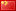## Programming Languages, Part B, HW4

570 2 2020年12月29日

5 1 投票

list-nth-mod: Incorrect implementation for n=0 (Result of (list-nth-mod (quote (-5)) 0) was expected to equal -5) [incorrect answer]

list-nth-mod: Exception for empty list (No exception raised) [incorrect answer]

#lang racket

(provide (all-defined-out)) ;; so we can put tests in a second file

(define (sequence low high stride)
(if (> low high)
null
(cons low
(sequence (+ low stride) high stride))))

(define (string-append-map xs suffix)
(map (lambda (x) (string-append x suffix)) xs))

(define (ith xs i)
(if (= i 0)
(car xs)
(ith (cdr xs) (- i 1))))

(define (list-nth-mod xs n)
(cond [(null? xs) error "list-nth-mod: empty list"]
[(< (car xs) 0) error "list-nth-mod: negative number"]
[#t (ith xs (remainder n (length xs)))]))

(define (stream-for-n-steps s n)
(if (= n 0)
null
(cons (car (s)) (stream-for-n-steps (cdr (s)) (- n 1)))))

(define (stream-maker f g args)
(letrec ([fn (lambda (x) (cons (f x) (lambda () (fn (g x)))))])
(lambda () (fn args))))

(define funny-number-stream
(stream-maker (lambda (x)
(if (= (remainder x 5) 0)
(- 0 x)
x))
(lambda (x)
(+ x 1))
1))

(define dan-then-dog
(stream-maker (lambda (x) x)
(lambda (x)
(if (string=? x "dan.jpg")
"dog.jpg"
"dan.jpg"))
"dan.jpg"))

(stream-maker (lambda (x) (cons 0 (car (x))))
(lambda (x) (cdr (x)))
s))

(define (cycle-lists xs ys)
(letrec ([fn (lambda (i j)
(cons (cons (ith xs i) (ith ys j))
(lambda ()
(fn (remainder (+ i 1) (length xs)) (remainder (+ j 1) (length ys))))))])
(lambda () (fn 0 0))))

(define (vector-assoc v vec)
(letrec ([check (lambda (i) (cond [(= i (vector-length vec)) #f]
[(pair? (vector-ref vec i)) (let ([p (vector-ref vec i)])
(cond [(equal? (car p) v) p]
[#t (check (+ i 1))]))]
[#t (check (+ i 1))]))])
(check 0)))

(define (cached-assoc xs n)
(letrec ([vec (make-vector n #f)]
[pos 0])
(lambda (v) (let ([cache (vector-assoc v vec)])
(cond [(equal? #f cache) (begin
(let ([ans (assoc v xs)])
(cond [(equal? ans #f) #f]
[#t (begin (vector-set! vec pos ans)
(set! pos (if (= pos (- n 1))
0
(+ pos 1)))
;(println pos)
ans)])))]
[#t (begin
;(println "found in cache")
cache)])))))

(define-syntax while-less
(syntax-rules (do)
[(while-less e1 do e2)
(letrec ([x e1]
[iter (lambda (y) (if (< y x)
(iter e2)
#t))])
(iter e2))]))

5 1 投票

2条留言（可选）如果您也有个人网站，不妨分享一下

2 评论kingno (@guest_2906)Chrome 87.0.4280.88 Mac OS X 10_13_6

2021年1月2日 10:37

sicp吗

Warning: error_log(/home/wwwroot/www.jxtxzzw.com/wp-content/plugins/spider-analyser/#log/log-3006.txt): failed to open stream: No such file or directory in /home/wwwroot/www.jxtxzzw.com/wp-content/plugins/spider-analyser/spider.class.php on line 2900